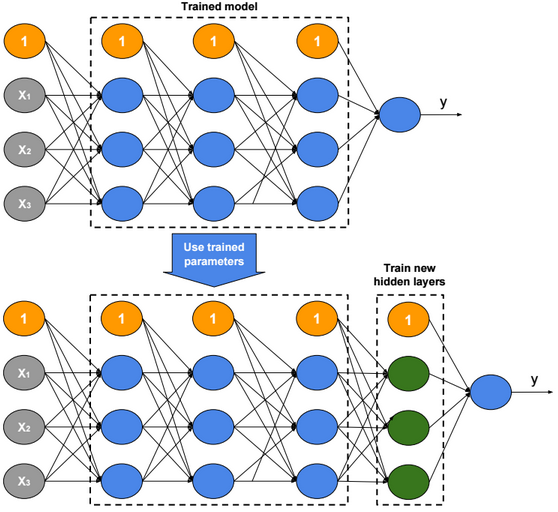# Deep Generative Models

Generative models is a class of unsupervised learning models.

Generative models estimate the density distribution of training data, and generate new samples from that distribution.

# PixelRNN

PixelRNN is a deep neural network that sequentially predicts/generates pixels in an image. The model estimates the density distribution of pixels by training a recurrent neural network.

$$p(image) = p(pixels) = \prod_{i=1}^{\#pixels} P(pixel_i|pixel_{i-1}, pixel_{i-2},…, pixel_0)$$

# PixelCNN

PixelRNN is accurate, but it is slow to train since RNN is hard to parallelize. PixelCNN is proposed to solve this problem. A mask is used to ensure convolution operation only uses previous pixels.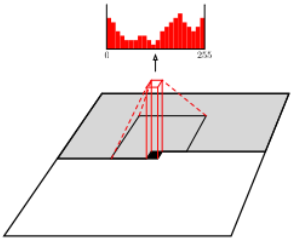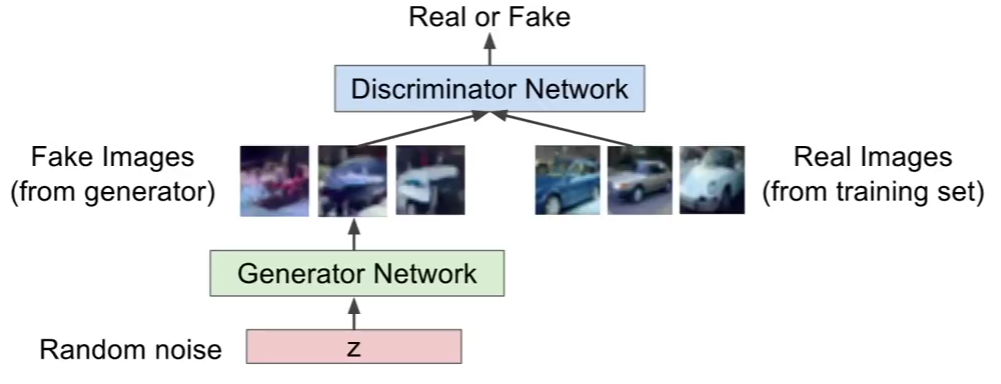GANs use two neural networks to generate real looking images. The first network generates fake images, and the second network distinguishes between real and fake images.

GANs require an iterative training process in which we train consecutively the discriminator and the generator. When training the generator we freeze discriminator weights. and when training the discriminator we freeze generator weights.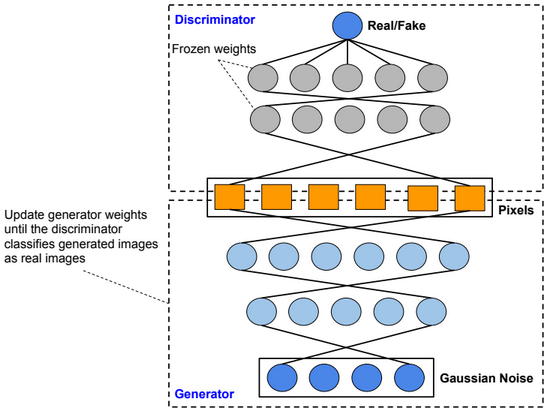Deep Convolutional GANs

Deep convolutional GANs (DC-GANs) use convolutional layers instead of dense layers.

Conditional GANs

Conditional GANs are an extension of the GAN framework. More details will be provided sooner.

An adversarial example is an example which has been modified very slightly in a way that is intended to cause a machine learning classifier to misclassify it.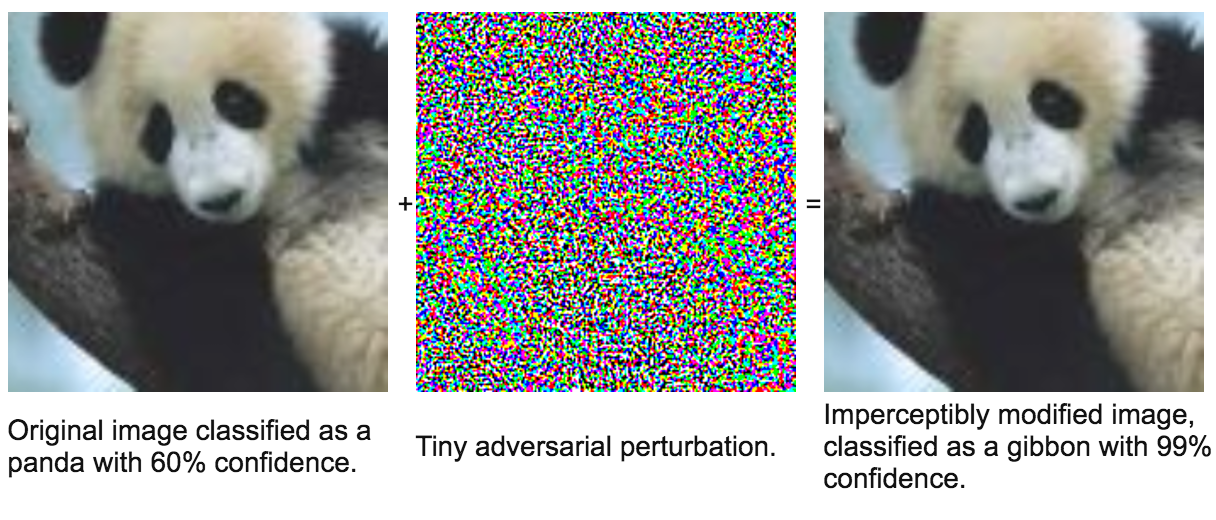Adversarial examples have the potential to be dangerous. For example, attackers could target autonomous vehicles by using stickers or paint to create an adversarial stop sign that the vehicle would interpret as a ÔÇśyieldÔÇÖ or other sign.

# Computer Vision Datasets

Famous computer Vision datasets are: MNIST, ImageNet, CIFAR-10(0), Places.

Below the error rate for deep neural networks trained on the ImageNet dataset.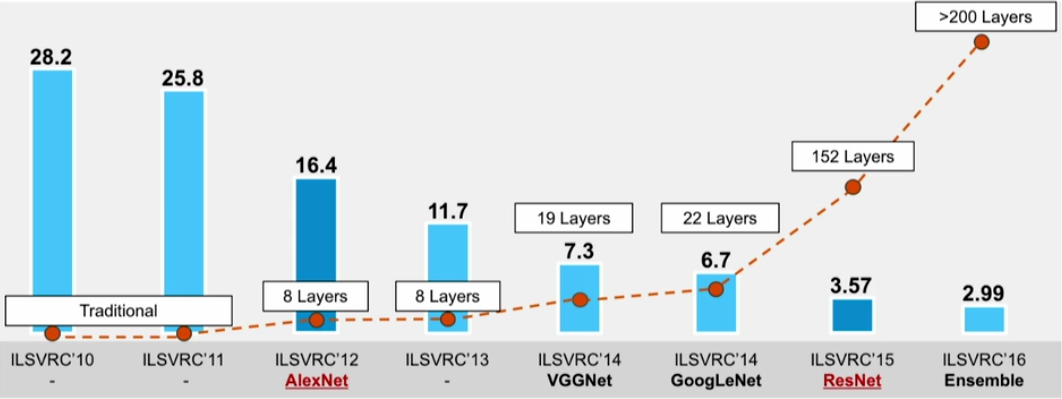# Convolution

A convolution is a neighborhood operation in which each output pixel is the weighted sum of neighboring input pixels. The matrix of weights is called the convolution kernel, also known as a filter.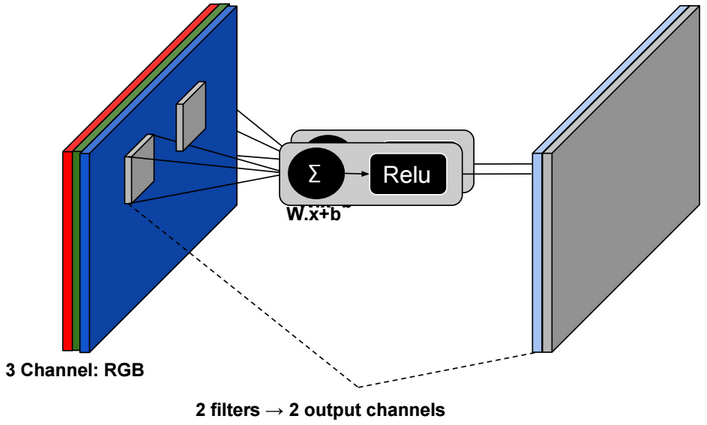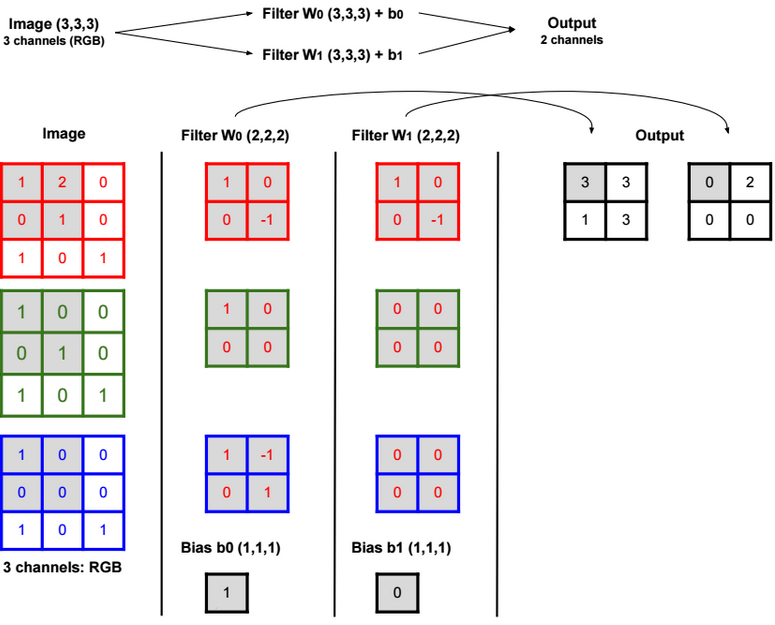Padding is basically adding rows or columns of zeros to the borders of an image input. It helps control the output size of the convolution layer. The formula to calculate the output size is: (N – F) / stride + 1.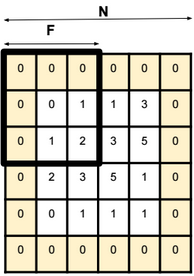For a 32x32x3 image and using 10 5×5 filters with stride 1 and pad 2, we get an output with size 32x32x10.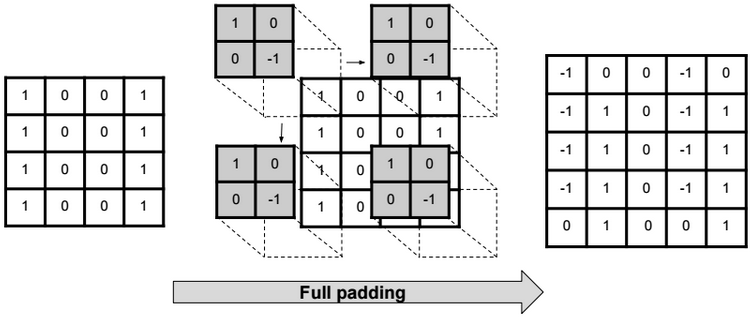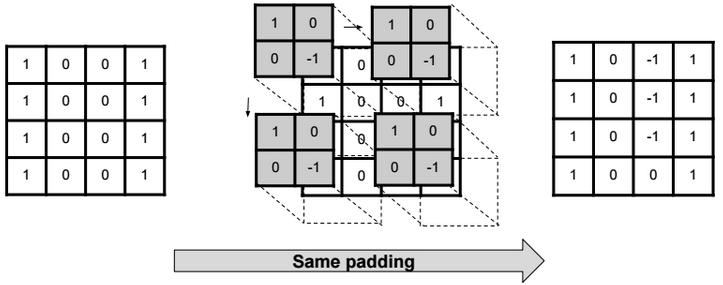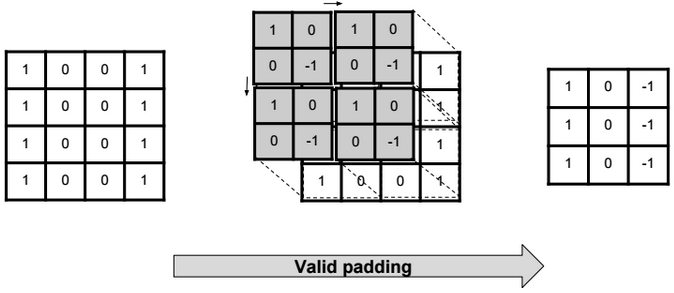# Transpose Convolution

Transpose Convolution (also called Deconvolution) is the reverse process of convolution.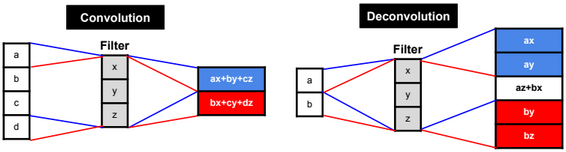The formula to calculate the output size is: stride.(input_w – 1) + ((input_w + 2.pad – kernel_w) mod stride) + kernel_w – 2.pad.

# Pooling

With pooling we reduce the size of the data without changing the depth.

Max pooling preserves edges.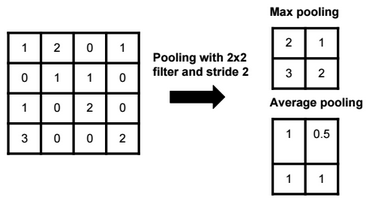The output size of a polling operation on a 8x8x10 representation using a 2×2 filter and with stride 2 is 4x4x10 (We can use the same formula: (N – F) / stride + 1).

Global Average pooling

Global Average pooling replaces all pixel values with one value per each channel. For example a Global Average Pooling of a 100×100 image with 3 channels (RGB) is a 1×1 image with 3 channels.

# Unpooling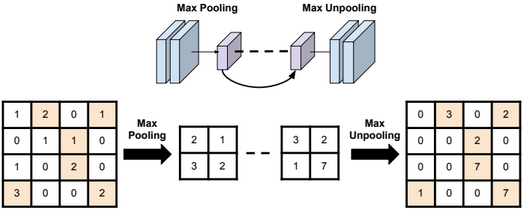Bed of nails unpooling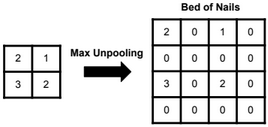Nearest neighbor unpooling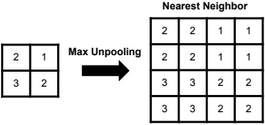# Architecture

In general the architecture of a convolution neural network is as below: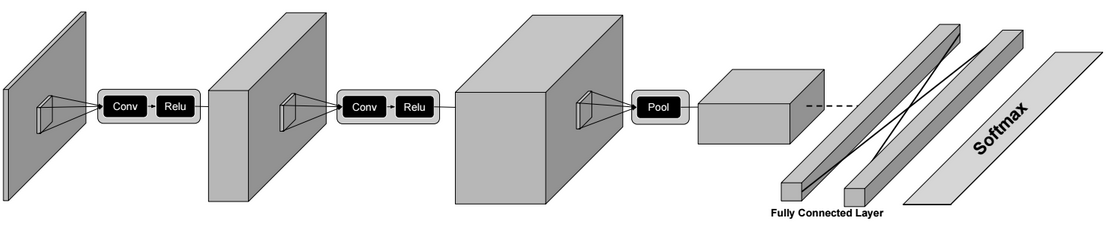Conv? Relu ? Conv ? Relu? Pool ? ů ? Conv ? Fully Connected Layer ? Softmax

Some well known CNN architectures are: AlexNet (8 layers), VGG (16-19 layers), GoogLeNet (22 layers) and ResNet (152 layers).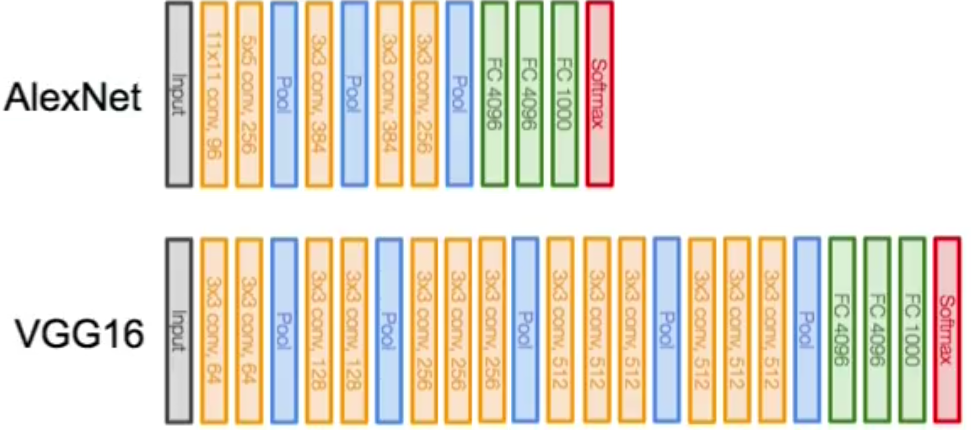For GoogleNet, the architecture is slightly different. It uses Inception modules which combine multiple parallel convolutions.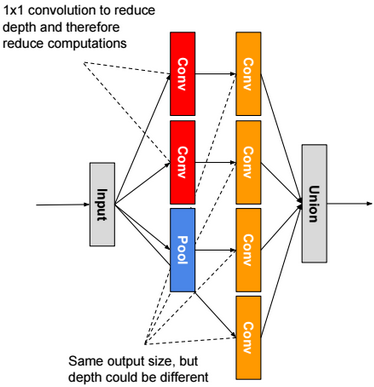For ResNet, we use residual blocks. The output of a residual block is the sum of the input X + the output of last convolution layer F(X). If weights are zeros, then the output of a residual block is the input X.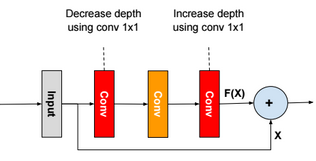There are other architectures like Network in Network, FractalNet, Densely Connected CNN, SqueezeNet.

Image Tagging

Basic classification of images.

Semantic Segmentation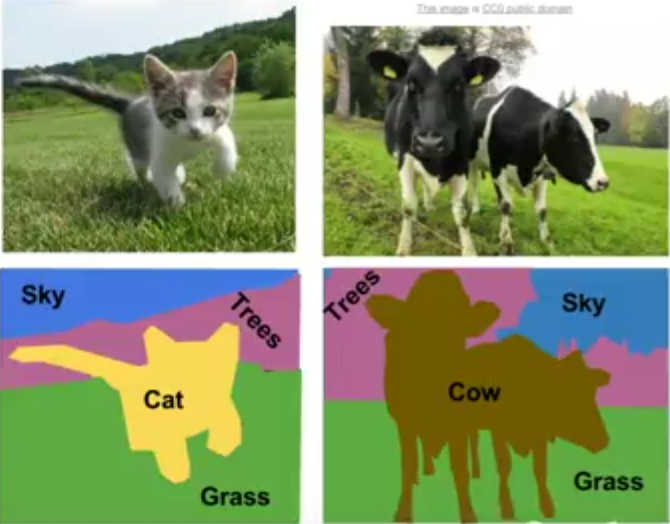Semantic segmentation is the task of assigning a class-label to each pixel in an image.

The general architecture of a CNN for this task is as follow: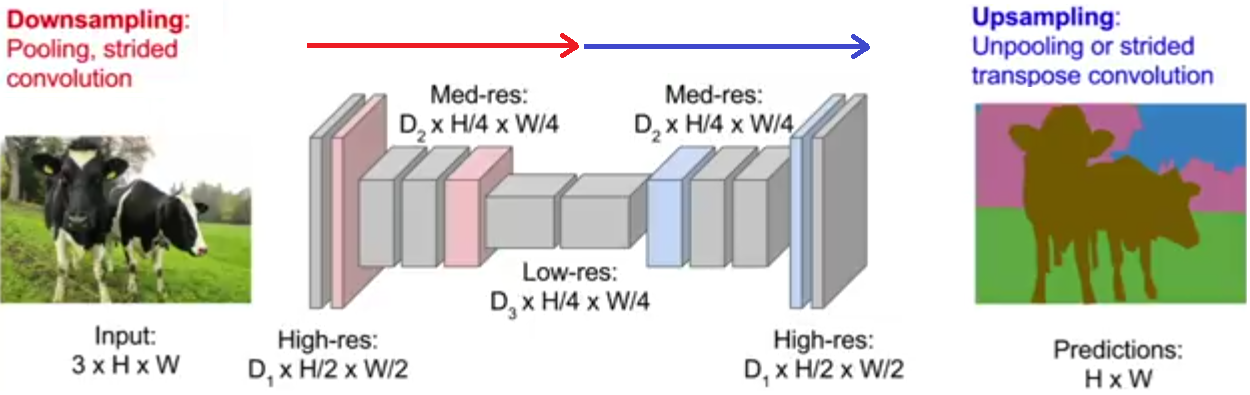In this task, we minimize the cross-entropy loss over every pixel.

Classification & Localisation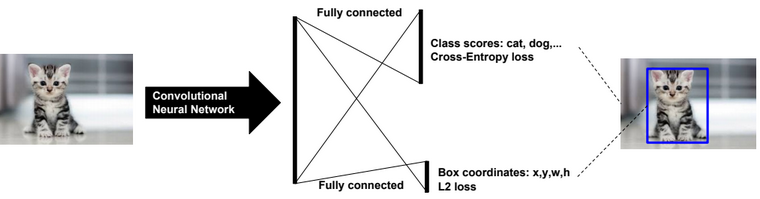Object Detection

Sliding window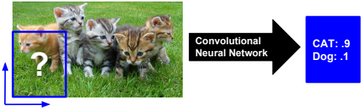Region proposals (selective search/R-CNN)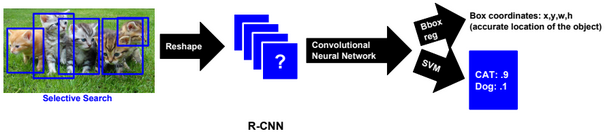Fast R-CNN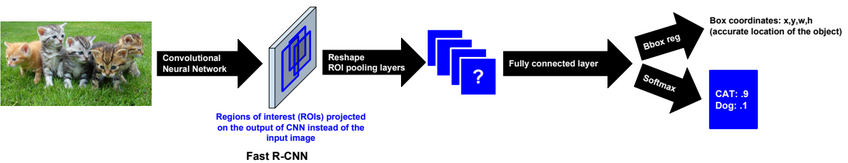Faster R-CNN

In Faster R-CNN we use and train a Region Proposal network instead of using selective search.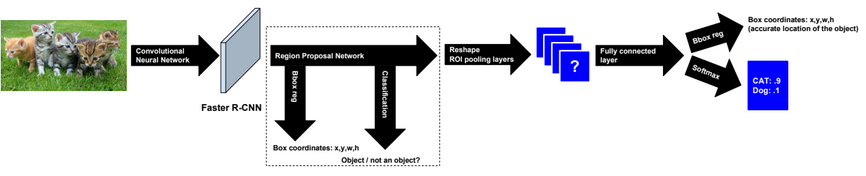Yolo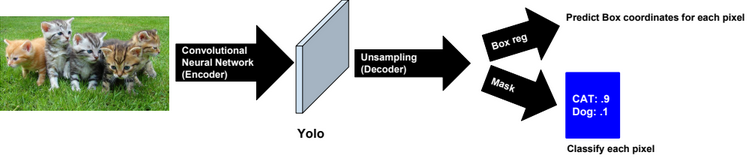Itĺs recommended to use Focal loss function when training the model.

Other methods

SSD

Instance Segmentation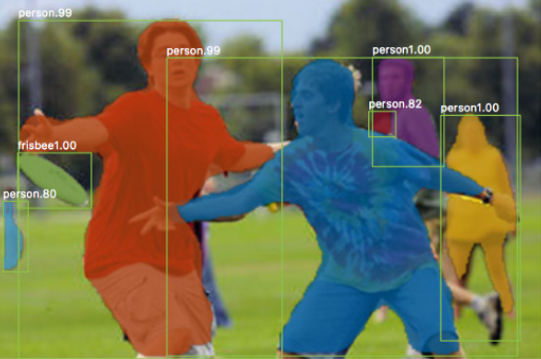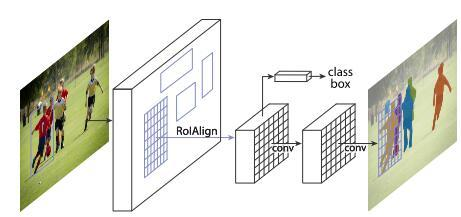# Inside CNN

To find the pixels in an input image that are important for a classification, we can use the Gradient Ascent or Feature Inversion algorithms.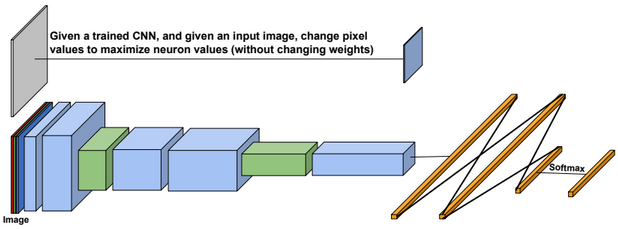# Architecture

RNNs are generally used for sequence modeling (e.g. language modeling, time series modeling,…).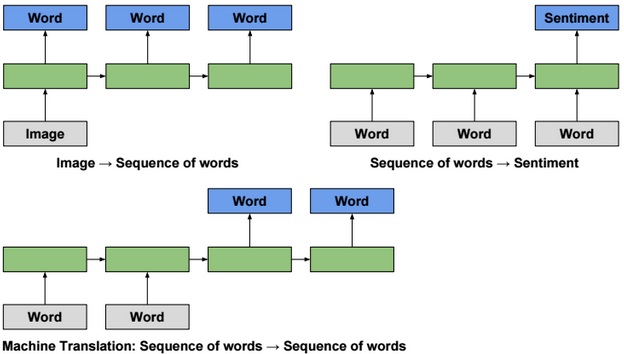Unfolding a RNN network (many to many scenario) could be presented as follow: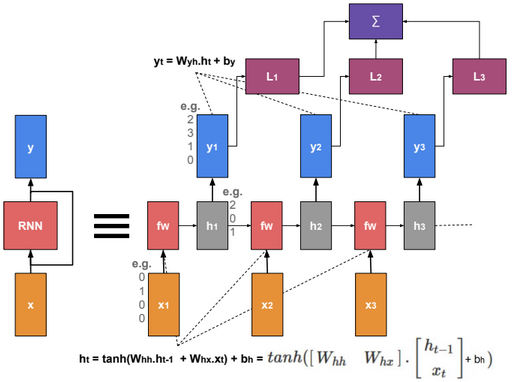When training the model, we need to find W that minimizes the sum of losses.

# Multilayer RNN

Multilayer RNN is a neural network with multiple RNN layers.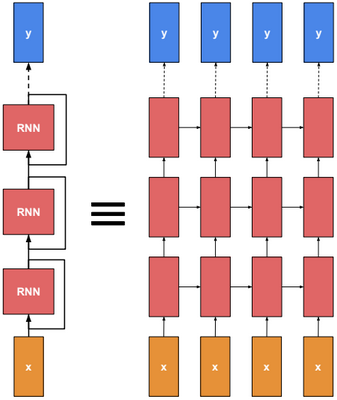# Backpropagation through time

To train a RNN, we need to calculate the gradient to update parameters. Instead of doing the derivations for the full network, we will focus only on one hidden unit.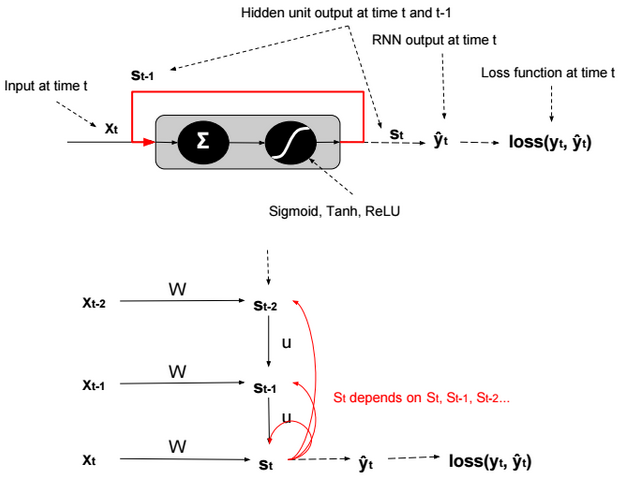We define $$s_t = g(W.x_t + U.s_{t-1} + b)$$. g is an activation function.

Using the chain rule we can find that:

$$\frac{\partial loss(y_t,?_t)}{\partial W} = \frac{\partial loss(y_t,?_t)}{\partial ?_t}.\frac{\partial ?_t}{\partial s_t}.\frac{\partial s_t}{\partial W} \\ = \frac{\partial loss(y_t,?_t)}{\partial ?_t}.\frac{\partial ?_t}{\partial s_t}.(\sum_{k=t}^0 \frac{\partial s_t}{\partial s_k}.\frac{\partial s_k}{\partial W})$$

The term in red in the equation is a product of Jacobians ($$\frac{\partial s_t}{\partial s_k} = \prod_{i=k+1}^{t} \frac{\partial s_{i}}{\partial s_{i-1}}$$).

$$\frac{\partial loss(y_t,?_t)}{\partial ?_t}.\frac{\partial ?_t}{\partial s_t}.(\sum_{k=t}^0 \color{red} {\frac{\partial s_t}{\partial s_k}}.\frac{\partial s_k}{\partial W})$$

Because the derivatives of activation functions (except ReLU) are less than 1, therefore when evaluating the gradient, the red term tends to converge to 0, and the model becomes more biased and captures less dependencies.

RNN is trained by backpropagation through time. When gradient is passed back through many time steps, it tends to vanish or to explode.

Gradient Clipping is a technique to prevent exploding gradients in very deep networks, typically Recurrent Neural Networks. There are various ways to perform gradient clipping, but the most common one is to normalize the gradients of a parameter vector when its L2 norm exceeds a certain threshold according to : $$gradients := gradients * \frac{threshold}{L2 norm(gradients)}$$.

# Long Short Term Memory (LSTM)

LSTM was introduced to solve the vanishing gradient problem.

In LSTM, there are 4 gates that regulate the state of a RNN model:

Write gate (i?[0,1]) (Input gate): Write to memory cell.

Keep gate (f?[0,1]) (Forget gate): Erase memory cell.

Gate gate (g?[-1,1]) (Update gate) How much to write to memory cell.

$$c_t$$ is called memory state at time t.

i,f,o,g,h,c are vectors having the same size. W is a matrix with size nxh.

The gate states are calculated using the following formula: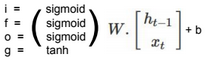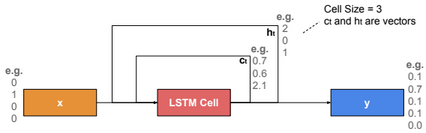The output $$h_t$$ is calculated using the following formulas:

$$c_t = f.c_{t-1} + i.g \\ h_t = o.tanh(c_t)$$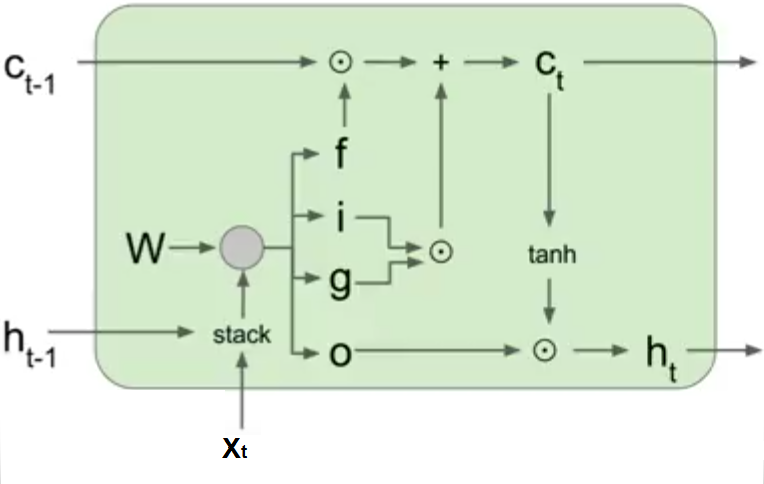# Gated Recurrent Unit (GRU)

The Gated Recurrent Unit is a simplified version of an LSTM unit with fewer parameters. Just like an LSTM cell, it uses a gating mechanism to allow RNNs to efficiently learn long-range dependency by preventing the vanishing gradient problem. The GRU consists of a reset and update gates that determine which part of the old memory to keep or update with new values at the current time step.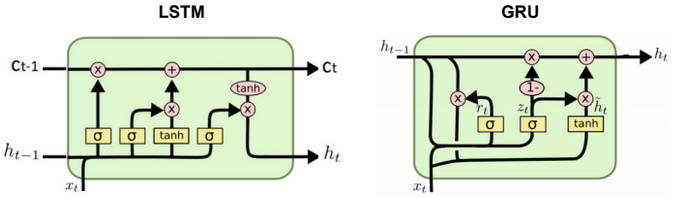# Neural Network

A neural network is a non-linear classifier (separator is not a linear function). It can also be used for regression.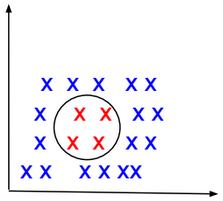A Shallow neural network is a one hidden layer neural network.

A Vanilla neural network is a regular neural network having layers that do not form cycles.

TensorFlow Playground is an interactive web interface for learning neural networks: http://playground.tensorflow.org.

# Computational Graph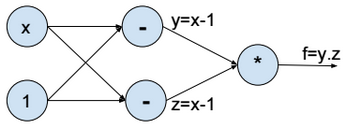Above the computational graph for the function $$f(x) = (x-1)^2$$.

Forward propagation

To minimize the function f, we assign a random value to x (e.g. x = 2), then we evaluate y, z, and f (forward propagation).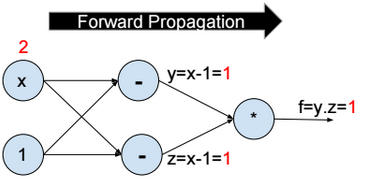Backward propagation

Then we compute the partial derivative of f with respect to x step by step (Backward propagation).

$$\frac{\partial f}{\partial x} = \frac{\partial f}{\partial y}*\frac{\partial y}{\partial x} + \frac{\partial f}{\partial z}*\frac{\partial z}{\partial x} = 2 \\ \frac{\partial f}{\partial y} = z = 1 \\ \frac{\partial f}{\partial z} = y = 1 \\ \frac{\partial y}{\partial x} = \frac{\partial z}{\partial x} = 1$$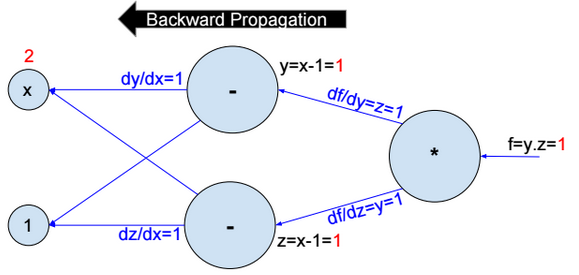Then we update $$x:= x – ?.\frac{\partial f}{\partial x}$$.

We repeat the operation until convergence.

# Activation functions

Activation functions introduce nonlinearity into models. The most used activation functions are:

Sigmoid

$$f(x) = \frac{1}{1+exp(-x)}$$

Sigmoid has a positive and non-zero centred output (sigmoid(0) ? 0.5).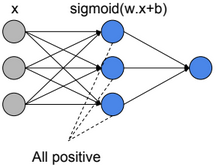When all activation units are positive, then weight update will be in the same direction (all positive or all negative updates) and that will cause a zigzag path during optimization.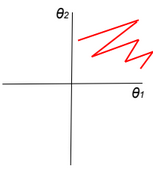$$z=?w_i.a_i+b \\ \frac{dL}{dw_i}=\frac{dL}{dz}.\frac{dz}{dw_i}=\frac{dL}{dz}.ai$$

If all ai>0, then the gradient will have the same sign as $$\frac{dL}{dz}$$ (all positive or all negative).

TanH

$$f(x) = \frac{2}{1+exp(-2x)} -1$$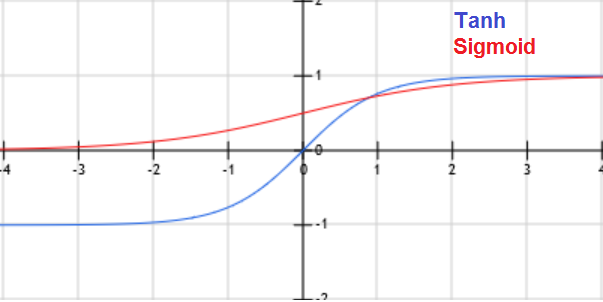When x is large, the derivative of the sigmoid or Tanh function is around zero (vanishing gradient/saturation).

ReLU (Rectified Linear Unit)

f(x) = max(0, x)

Leaky ReLU

f(x) = max(0.01x, x)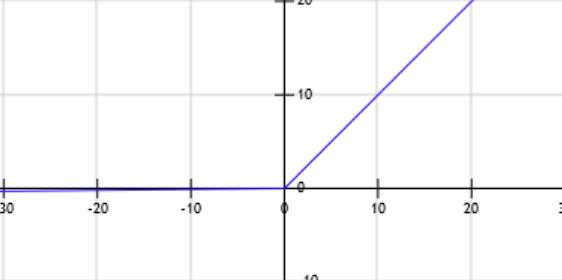Leaky Relu was introduced to fix the ôDying Reluö problem.

$$z=?w_i.a_i+b \\ f=Relu(z) \\ \frac{dL}{dw_i}=\frac{dL}{df}.\frac{df}{dz}.\frac{dz}{dw_i}$$

When z becomes negative, then the derivative of f becomes equal to zero, and the weights stop being updated.

PRelu (Parametric Rectifier)

f(x) = max(?.x, x)

ELU (Exponential Linear Unit)

f(x) = {x if x>0 otherwise ?.(exp(x)-1)}

Other activation functions: Maxout

# Cost function

$$J(?) = \frac{1}{m} \sum_{i=1}^{m} loss(y^{(i)}, f(x^{(i)}; ?))$$

We need to find ? that minimizes the cost function: $$\underset{?}{argmin}\ J(?)$$

# Neural Network Regression

Neural Network regression has no activation function at the output layer.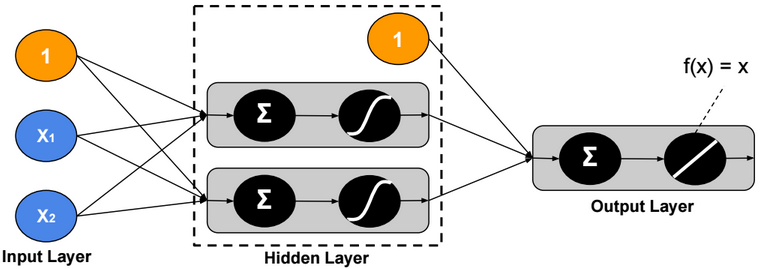L1 Loss function

$$loss(y,?) = |y – ?|$$

L2 Loss function

$$loss(y,?) = (y – ?)^2$$

Hinge loss function

Hinge loss function is recommended when there are some outliers in the data.

$$loss(y,?) = max(0, |y-?| – m)$$

# Two-Class Neural Network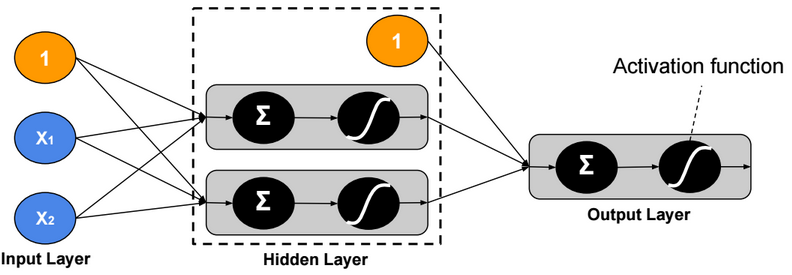Binary Cross Entropy Loss function

$$loss(y,?) = – y.log(?) – (1-y).log(1 – ?)$$

# Multi-Class Neural Network – One-Task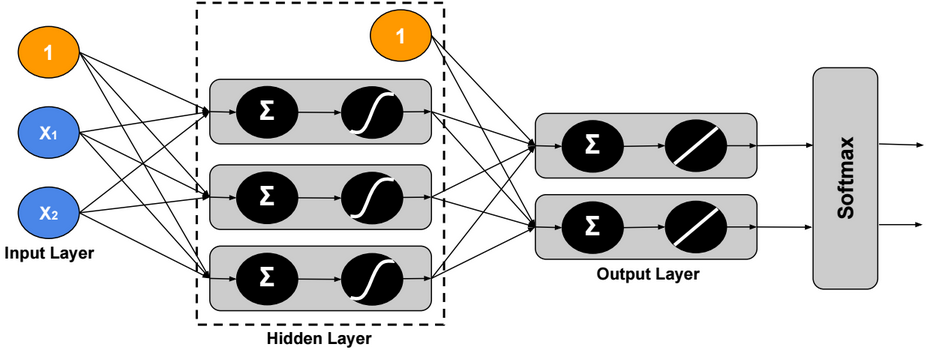Using Softmax, the output ? is modeled as a probability distribution, therefore we can assign only one label to each example.

Cross Entropy Loss function

$$loss(Y,\widehat{Y}) = -\sum_{j=1}^c Y_{j}.log(\widehat{Y}_{j})$$

Hinge Loss (SVM) function

$$y = \begin{bmatrix} 1 \\ 0 \\ 0 \end{bmatrix},\ ? = \begin{bmatrix} 2 \\ -5 \\ 3 \end{bmatrix} \\ loss(y,?) = \sum_{c?1} max(0, ?_c – ?_1 + m)$$

For m = 1, the sum will be equal to 2.

# Multi-Class Neural Network – Multi-Task

In this version, we assign multiple labels to each example.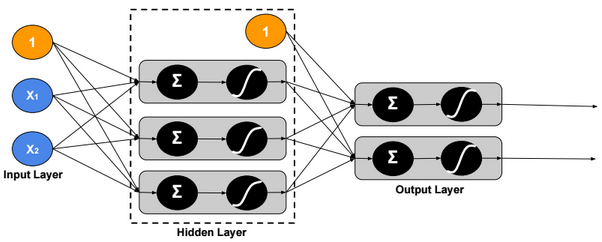Loss function

$$loss(Y,\widehat{Y}) = \sum_{j=1}^c – Y_j.log(\widehat{Y}_j) – (1-Y_j).log(1 – \widehat{Y}_j)$$

# Regularization

Regularization is a very important technique to prevent overfitting.

DropoutFor each training example, ignore randomly p activation nodes of each hidden layer. p is called dropout rate (p?[0,1]). When testing, scale activations by the dropout rate p.

Inverted Dropout

With inverted dropout, scaling is applied at the training time, but inversely. First, dropout all activations by dropout factor p, and second, scale them by inverse dropout factor 1/p. Nothing needs to be applied at test time.

Data Augmentation

As a regularization technique, we can apply random transformations on input images when training a model.

Early stopping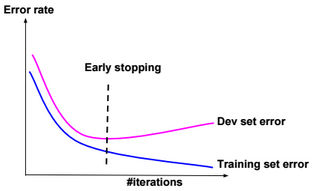Stop when error rates decreases on training data while it increases on dev (cross-validation) data.

L1 regularization

$$J(?) = \frac{1}{m} \sum_{i=1}^{m} loss(y^{(i)}, f(x^{(i)}; ?)) \color{blue} { + ? .\sum_{j} |?_j|}$$

? is called regularization parameter

L2 regularization

$$J(?) = \frac{1}{m} \sum_{i=1}^{m} loss(y^{(i)}, f(x^{(i)}; ?)) \color{blue} { + ? .\sum_{j} ?_j^2}$$

Lp regularization

$$J(?) = \frac{1}{m} \sum_{i=1}^{m} loss(y^{(i)}, f(x^{(i)}; ?)) \color{blue} { + ? .\sum_{j} ?_j^p}$$

For example, if the cost function $$J(?)=(?_1 – 1)^2 + (?_2 – 1)^2$$, then the $$L_2$$ regularized cost function is $$J(?)=(?_1 – 1)^2 + (?_2 – 1)^2 + ? (?_1^2 + ?_2^2)$$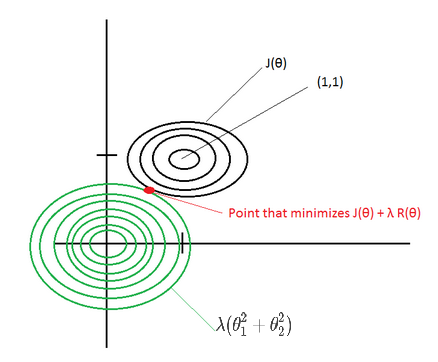If ? is large, then the point that minimizes the regularized J(?) will be around (0,0) –> Underfitting.

If ? ~ 0, then the point that minimizes the regularized J(?) will be around (1,1) –> Overfitting.

Elastic net

Combination of L1 and L2 regularizations.

# Normalization

Gradient descent converges quickly when data is normalized Xi ? [-1,1]. If features have different scales, then the update of parameters will not be in the same scale (zig-zag).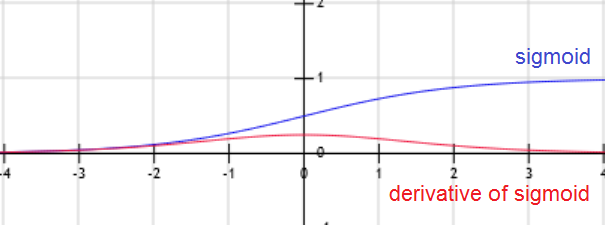For example, if the activation function g is the sigmoid function, then when W.x+b is large g(W.x+b) is around 1, but the derivative of the sigmoid function is around zero. For this reason the gradient converges slowly when the W.x+b is large.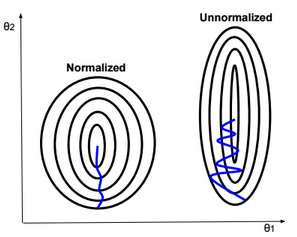Below some normalization functions.

ZScore

$$X:= \frac{X – ?}{?}$$

MinMax

$$X:= \frac{X – min}{max-min}$$

Logistic

$$X:= \frac{1}{1+exp(-X)}$$

LogNormal

$$X:= \frac{1}{?\sqrt{2?}} \int_{0}^{X} \frac{exp(\frac{-(ln(t) – ?)^2}{2?^2})}{t} dt$$

Tanh

$$X:= tanh(X)$$

# Weight Initialization

Weight initialization is important because if weights are too big then activations explode. If weights are too small then gradients will be around zero (no learning).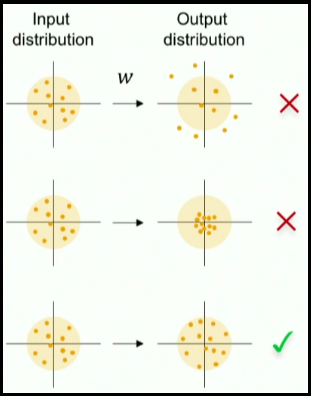When we normalize input data, we make the mean of the input features equals to zero, and the variance equals to one. To keep the activation units normalized too, we can initialize the weights $$W^{(1)}$$ so $$Var(g(W_{j}^{(1)}.x+b_{j}^{(1)}))$$ is equals to one.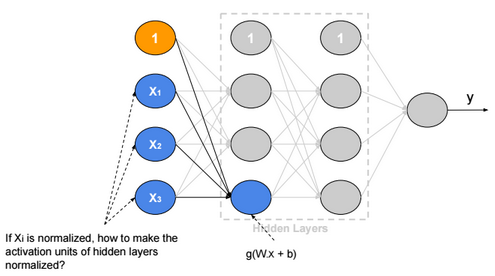If we suppose that g is Relu and $$W_{i,j}, b_j, x_i$$ are independent, then:

$$Var(g(W_{j}^{(1)}.x+b_{j}^{(1)})) = Var(\sum_{i} W_{i,j}^{(1)}.x_i+b_{j}^{(1)}) =\sum_{i} Var(W_{i,j}^{(1)}.x_i) + 0 \\ = \sum_{i} E(x_i)^2.Var(W_{i,j}^{(1)}) + E(W_{i,j}^{(1)})^2.Var(x_i) + Var(W_{i,j}^{(1)}).Var(x_i) \\ = \sum_{i} E(x_i)^2.Var(W_{i,j}^{(1)}) + E(W_{i,j}^{(1)})^2.Var(x_i) + Var(W_{i,j}^{(1)}).Var(x_i) \\ = \sum_{i} 0 + 0 + Var(W_{i,j}^{(1)}).Var(x_i) = n.Var(W_{i,j}^{(1)}).Var(x_i)$$

Xavier initialization

If we define $$W_{i,j}^{(1)} ? N(0,\frac{1}{\sqrt{n}})$$, then the initial variance of activation units will be one (n is number of input units).

We can apply this rule on all weights of the neural network.

# Batch Normalization

Batch normalization is a technique to provide any layer in a Neural Network with normalized inputs. Batch Normalization has a regularizing effect.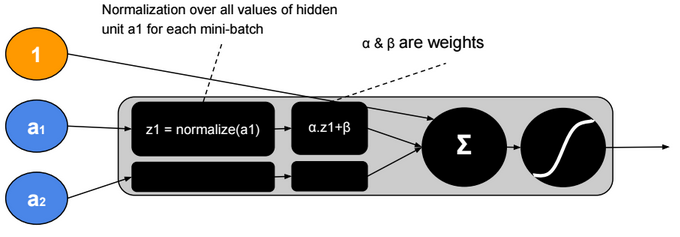After training, ? will converge to the standard deviation of the mini-batches and ? will converge to the mean. The ?, ? parameters give more flexibility when shifting or scaling is needed.

# Hyperparameters

Neural network hyperparameters are:

• Learning rate (?) (e.g. 0.1, 0.01, 0.001,…)
• Number of hidden units
• Number of layers
• Mini-bach size
• Momentum rate (e.g. 0.9)
• Adam optimization parameters (e.g. ?1=0.9, ?2=0.999, ?=0.00000001)
• Learning rate decay

# Local Minimum

The probability that gradient descent gets stuck in a local minimum in a high dimensional space is extremely low. We could have a saddle point, but itĺs rare to have a local minimum.

# Transfer Learning

Transfer Learning consists in the use of parameters of a trained model when training new hidden layers of an extended version of that model.